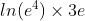## Ln Base

Solve the shown logarithm:Hint
The logarithm of $$x$$ to the Base $$b$$ is defined by
$$log_b(x)=c$$$where $$b^c=x$$ . Hint 2 Special definitions when $$b=e$$ or $$b=10$$ are: • ln $$x$$ → Base = $$e$$ • log $$x$$ → Base = 10 The logarithm of $$x$$ to the Base $$b$$ is defined by $$log_b(x)=c$$$
where $$b^c=x$$ . Special definitions when $$b=e$$ or $$b=10$$ are:
• ln $$x$$ → Base = $$e$$
• log $$x$$ → Base = 10

Since the natural log has a default base of $$e$$ :
$$ln(e^4) \times 3e=ln_e(e^4) \times 3e=(4)\cdot 3e=12e$$\$
Since Euler’s number, $$e$$ , is a mathematical constant approximately equal to 2.718, another answer is: $$12 \times 2.718=32.6$$ .
12 $$e$$ or 32.6